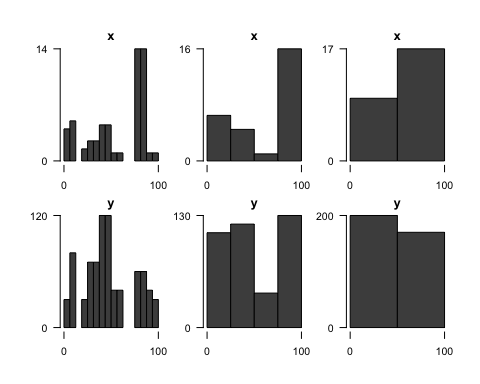# Introduction to linbin

### Event Tables

Event tables are custom data frames used throughout linbin to store and manipulate linearly referenced data. Each row includes an event’s endpoints from and to (which can be equal, to describe a point, or non-equal, to describe a line) and the values of any variables measured on that interval. The built in simple data frame is a small but not so simple event table with line and point events, gaps, overlaps, and missing values.

e <- simple
from to x y z factor
0 0 1.0 60 1.9 a
0 10 4.0 30 0.3 a
10 10 1.0 50 0.9 b
20 50 1.5 30 NA NA
30 60 1.0 40 0.2 a
40 50 2.0 50 1.5 b
75 85 2.0 50 1.4 a
75 85 12.0 10 0.4 a
90 90 1.0 40 0.8 b
90 90 NA NA 1.2 NA
95 100 1.0 30 0.6 a

The central purpose of this package is to summarize event variables over sampling intervals, or “bins”, and plot the results. Batch binning and plotting allows the user to quickly visualize multivariate data at multiple scales, useful for identifying patterns within and between variables, and investigating the influence of scale of observation on data interpretation. For example, using the simple event table above, we can compute sequential bins fitted to the range of the events with seq_events(), compute bin statistics from the events falling within each bin with sample_events(), and plot the results with plot_events().

bins <- seq_events(event_range(e), length.out = 5)
e.bins <- sample_events(e, bins, list(mean, "x"), list(mean, "y", by = "factor", na.rm = TRUE))
from to x y.a y.b y.NA
0 20 2.50 30 50 NA
20 40 1.25 40 NA 30
40 60 1.50 40 50 30
60 80 7.00 30 NA NA
80 100 NA 30 40 NaN
plot_events(e.bins, xticks = axTicks, border = par("bg"))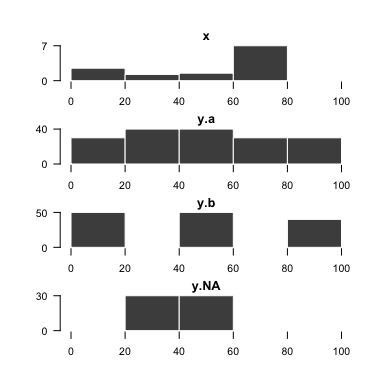Below, we describe in more detail the core steps and functions of a typical linbin workflow.

### Create an Event Table : events(), as_events(), read_events()

Event tables can be created from scratch with events():

events(from = c(0, 15, 25), to = c(10, 30, 35), x = 1, y = c('a', 'b', 'c'))
>   from to x y
> 1    0 10 1 a
> 2   15 30 1 b
> 3   25 35 1 c

Coerced from existing objects with as_events():

as_events(1:3) # vector
>   from to
> 1    1  2
> 2    2  3
as_events(cbind(1:3, 2:4)) # matrix
>   from to
> 1    1  2
> 2    2  3
> 3    3  4
as_events(data.frame(start = 1:3, x = 1, stop = 2:4), "start", "stop") # data.frame
>   from x to
> 1    1 1  2
> 2    2 1  3
> 3    3 1  4

Or read directly from a text file with the equivalent syntax read_events(file, from.col, to.col).

### Design the Bins : event_range(), event_coverage(), event_overlaps(), fill_event_gaps(), seq_events(), …

seq_events() generates groups of sequential bins fitted to the specified intervals. Different results can be obtained by varying to what, and how, the bins are fitted. The simplest approach to fitting bins to data is to use the event_range(), the interval bounding the range of the data. An alternative is the event_coverage(), the intervals over which the number of events remains greater than zero — the inverse of event_gaps(). For finer control, event_overlaps() returns the number of overlapping events on each interval. fill_event_gaps() fills gaps less than a maximum length to prevent small gaps in coverage from being preserved in the bins. Using the simple event table as an example: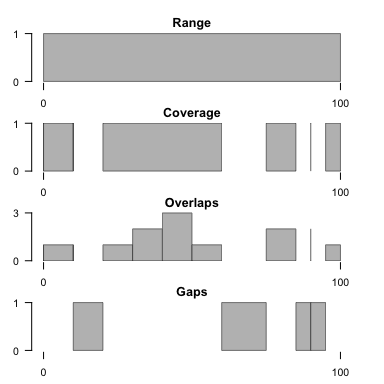These various metrics can be used to generate bins serving particular needs. Some strategies are listed below as examples, and applied to the built in elwha event table to plot longitudinal profiles of mean wetted width throughout the Elwha River (Washington, USA).

e <- elwha
1. Minimally flatten the event data to 1-dimensions by using bins spanning the intervals of event overlap. In the absence of overlaps, these are equal to the events themselves.
bins <- event_overlaps(e)
e.bins <- sample_events(e, bins, list(weighted.mean, "mean.width", "unit.length"),
scaled.cols = "unit.length")
plot_events(e.bins, data.cols = "mean.width", col = "grey", border = "#666666",
ylim = c(0, 56), main = "", oma = rep(0, 4), mar = rep(0, 4),
xticks = NA, yticks = NA)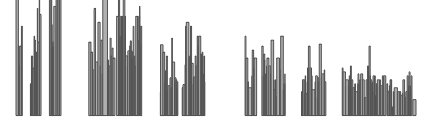1. Divide the range of the data into equal-length bins. A conventional approach that yields regular bins, but ignores the presence of any gaps in the data.
bins <- seq_events(event_range(e), length.out = 33)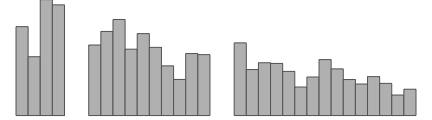1. Divide the coverage of the data into equal-coverage bins. By straddling gaps, each bin contains an equal length of sampled data, minimizing sampling bias.
bins <- seq_events(event_coverage(e), length.out = 20)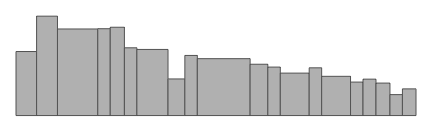1. Vary the lengths of bins locally to fit the coverage of the data. By explicitly preserving gaps, this strategy minimizes edge effects and can ensure that the bin endpoints correspond to important features (e.g., tributary confluences in river networks).
e.filled <- fill_event_gaps(e, max.length = 1) # fill small gaps first
bins <- seq_events(event_coverage(e.filled), length.out = 20, adaptive = TRUE)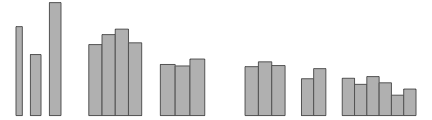### Sample Events at Bins : cut_events(), sample_events()

sample_events() computes event table variables for the specified sampling intervals, or “bins”. The sampling functions to use are passed as a series of list arguments in the format list(FUN, data.cols.first, ..., by = group.cols, ...), where:

• FUN — The first element is the function to use. It must compute a single value from one or more vectors of the same length. Functions commonly used on single numeric variables include sum(), mean(), sd(), min() and max(). Functions commonly used on multiple variables include weighted.mean().

• data.cols.first — The next (unnamed) element is a vector specifying the event column names or indices to pass in turn as the first argument of the function. Names are interpreted as regular expressions (regex) matching full column names.

• ... — Any additional unnamed elements are vectors specifying event columns to pass as the second, third, … argument of the function.

• by = group.cols — The first element named by is a vector of event column names or indices used as grouping variables.

• ... — Any additional named arguments are passed directly to the function unchanged.

Binning begins by cutting events at bin endpoints using cut_events(). When events are cut, event variables can be rescaled by the relative lengths of the resulting event segments by naming them in the argument scaled.cols. This is typically the desired behavior when computing sums, since otherwise events will contribute their full total to each bin they intersect.

With the simple event table as an example:

e <- simple
bins <- seq_events(event_range(e), length.out = 1)

Compute the sum of x and y, ignoring NA values and rescaling both at cuts:

e.bins <- sample_events(e, bins, list(sum, c('x', 'y'), na.rm = TRUE), scaled.cols = c('x', 'y'))
from to x y
0 100 25.5 330

Compute the mean of x with weights y, ignoring NA values:

e.bins <- sample_events(e, bins, list(weighted.mean, 'x', 'y', na.rm = TRUE))
from to x
0 100 1.954546

Paste together all unique values of factor (using a custom function):

fun <- function(x) paste0(unique(x), collapse = '.')
e.bins <- sample_events(e, bins, list(fun, 'factor'))
from to factor
0 100 a.b.NA

### Plot the Binned Data : plot_events()

plot_events() plots an event table as a grid of bar plots. Given a grouping variable for the rows of the event table (e.g., groups of bins of different sizes), and groups of columns to plot, bar plots are drawn in a grid for each combination of event and column group. If a column group contains multiple event columns, they are plotted together as stacked bars. Point events are drawn as thin vertical lines. Overlapping events are drawn as overlapping bars, so it is better to use sample_events() with groups of non-overlapping bins to flatten the data to 1-dimensions before plotting. Many arguments are available to control the appearance of the plot grid. The default output looks like the following:

e <- simple
bins <- seq_events(event_range(e), length.out = c(16, 4, 2)) # appends a "group" column
e.bins <- sample_events(e, bins, list(sum, c('x', 'y'), na.rm = TRUE))
plot_events(e.bins, group.col = 'group')